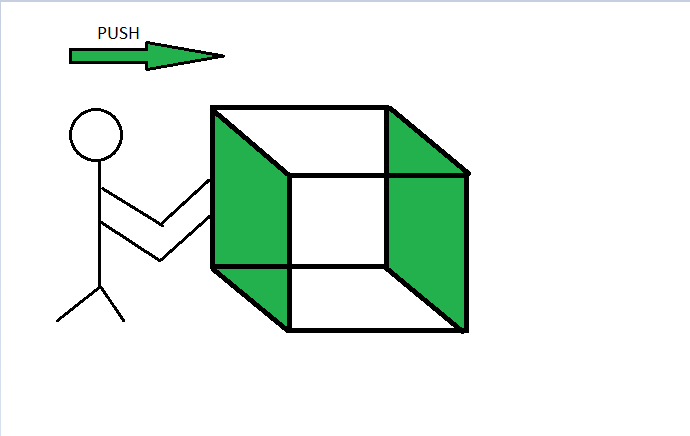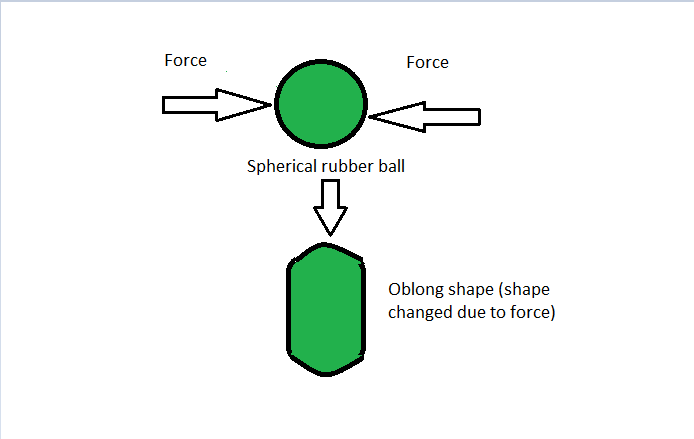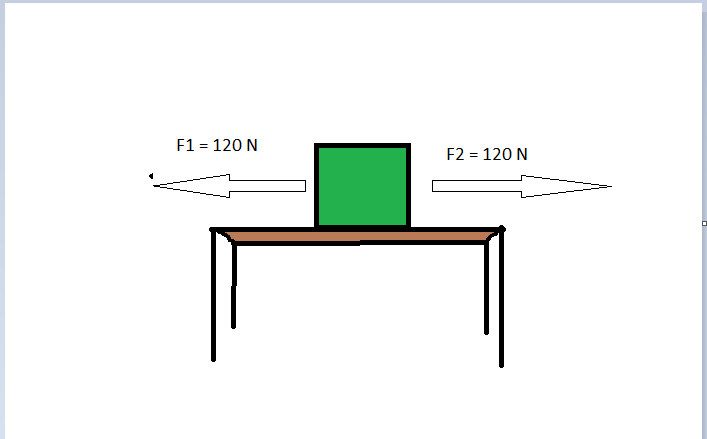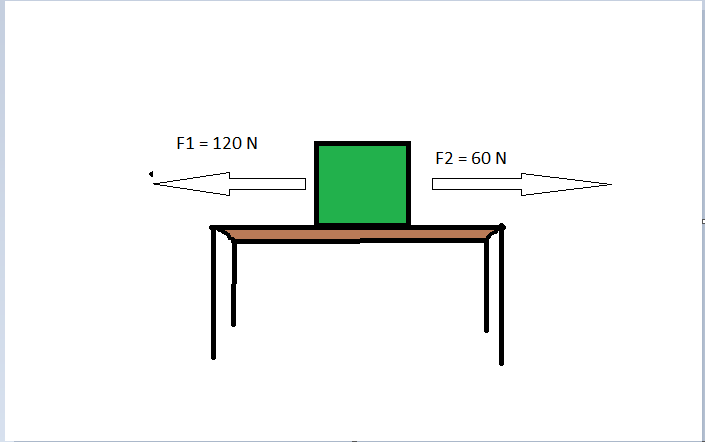# Force – Definition, Effects, Types, Sample Problems

• Difficulty Level : Easy
• Last Updated : 27 Jul, 2022

In our everyday life, it is observed that some effort is required to put an object in a rest state into motion or to stop a moving body. it is experiencing this as a muscular effort and say that we must push or pull on an object to change its state of motion. The concept of force is based on this push, hit, or pull only.

Hence, Force can be defined as a push, pull, or hit on any object that brings a change in its state of motion. A force is exerted on one object by another.

e.g,: When a box is pushed, it moves forward and changes its state of motion, hence we can say that when a force was applied to the box in the form of a push, it changed its state from rest to motion. Similarly, suppose a box is tied with a rope and is pulled by a person, then the box will change its state of rest and will start moving in the direction of the pull. This is another example of Force.A force is being applied to the box in the form of a push

Force can also change the shape and size of any object. The greater the force is applied, the more the changes will be observed in the shape and size of any object.

e.g:, When a force is applied to a spherical rubber ball, it changes its shape from spherical to oblong.

Another example can be of a rubber spring, as when a force is applied to a spring in the form of a pull, it gets expanded and hence changed its shape.Due to the application of force, the shape of a ball got changed

In this way, the force has many applications in our everyday lives such as the force due to one’s foot pushing on the pedal when riding a bicycle, the force applied of the bat by the ball. A general case for the existence of the normal force is when a person stands on the ground i.e. the ground exerts a force on the person that is equal in magnitude to the person’s weight.

## Effects of Force

From the above examples, various effects of force can be summed as below:

• It can change the state of motion of a body.
• It can change the direction of the moving body.
• It can change the size or shape of a body.
• It can accelerate the speed of the moving body.
• It can slow down the speed of the moving body.

## Types of Forces

There are two types of Forces depending upon whether the effect of force is seen or felt or not. These are as follows:

1) Balanced force

2) Unbalanced force

### Balanced Forces

The equal and opposite force acting on an object that does not change the state of rest or of motion of that object is known as the Balanced force

The forces acting on an object are said to be balanced (or if there are no forces acting on it) when the object is not moving stays still or the object which is moving continuously with the same speed and in the same direction.  Therefore, the types of forces in which the resultant of all the forces acting on the object is zero are known as balanced forces.

e.g.:, When a wooden block is kept on a table and equal forces are applied on both sides of the block then the block will not move in any direction.Balanced forces acting on the block

Clearly, the forces are balanced as a force of 120 N is applied to the left of the block as well as to the right of the block. The net force acting on the box must be equal to the difference between the two forces F1 and F2:

F = F1 – F2 = 120 N – 120 N = 0 N.

Since the resultant/net force is zero hence, the block will not change its state of rest.

Another example can be of a box placed on the rough surface, So if the box is pushed with a small force, the box do not change its position or remains at rest due to the frictional force acting in a direction opposite to the push it. This means the box balances the pushing force acting on it with the frictional force between the box and surface, of the same amount and therefore the box does not move.

### Unbalanced Forces

The unequal and opposite force acting on an object, that tends to move the object in the direction of larger force applied is known as the unbalanced force

The forces acting on an object are said to be unbalanced forces when the net force on the object is not zero. When the net forces acting on then the object results in an unbalanced force, then due to which the force accelerates the object. This means that a net force acting on the object can either change the direction or magnitude of its velocity.

e.g.:, Consider the same case of the box as described in balanced force, with a difference that the two forces applied are of different magnitudes on the opposite sides of the block, so the block moves in the direction of the larger force.Balanced forces acting on the block

Clearly, the forces are unbalanced as a force of 120 N is applied to the left of the block while a force of 60 N is applied to the right of the block.

The net force acting on the box must be equal to the difference between the two forces F1 and F2:

F = F1 – F2 = 120 N – 60 N = 60 N.

Since F1 is the greater force hence, the block will move in the direction of F1.

So, it is concluded that an object moves with a constant speed when all the forces acting on the object are balanced and there is no net external force acting on it.

When an unbalanced force is applied to the object, there will be a change either in its speed or in the direction of its motion.

Thus, to accelerate the motion of an object, an unbalanced force is required. And the change in its speed (or in the direction of motion) would continue as long as this unbalanced force is applied.

## Units of Force

As force has both magnitudes and direction, so it is a Vector quantity.

The direction of the force can simply be represented with the help of an arrow whereas to represent the magnitude of the force, some measuring units are required. So the standard units of force are as follows:

• Force can be expressed in Newton (N), in the standard international system of the unit (SI unit).
• While, in dyne, in the centimeter gram second system of unit (CGS unit).

Note: In the SI base unit, Newton is equivalent to Kg m/s2

## Representation of Force

Force is denoted by the symbol (F). The quantity of force can be expressed by the vector product of mass (m) and acceleration (a). So the mathematical representation of the force can be written as follows:

F = m × a

where, F is the force, m is the mass of the object and a is the acceleration obtained.

Now, since acceleration is the change in the velocity of the moving body, hence other mathematical representation of the force can be written as follows:

F = m × a

Since,

a = (v-u)/t

So,

F = m × (v-u)/t

where, u and v are the initial and final velocities respectively and t is the time taken.

## Solved Problems

Problem 1: Suppose one have a box of mass 2 kg. How much force should be applied to the box so that it should travel with the acceleration of 8 m/s2?

Solution:

Given that,

The mass of the box, m = 2 kg and

The acceleration of the box a = 8m/s2.

The formula to calculate the force is:

F = m × a

So on putting the value of ‘m’ and ‘a’ in the above equation as:

F = 2 kg × 8 m/s2

= 16 kg m/s2 or 16 N.

Problem 2: A ball of mass 2 kg is moving with the initial speed of 5 m/s and then suddenly comes to rest in 0.02 sec. Calculate the force that changed the state of the moving ball.

Solution:

Given that,

The initial velocity of the ball, u = 5 m/s,

The final velocity of the ball, v = 0 m/s,

The time taken, t = 0.02 s.

The formula to calculate the force is:

F = m × a

where, m is the mass and a is the acceleration.

and the formula to calculate the acceleration is:

a = (v-u)/t

where, t is the time taken, v and u are the initial and final velocities of the ball.

Substitute (v-u)/t for a in the first expression of force as:

F = m × (v-u)/t

Now, putting the given values in the above expression to calculate the force.

a = (0-5)/0.02 = -250 m/s2  [-ive sign simply represents the negative acceleration known as retardation ]

on putting this value in eq 1, we get

F = 2 kg × (0 m/s – 5 m/s)/0.02 s = 2 kg × -250 m/s2 = 500 N.

Problem 3: If a block is placed on a wooden table such that on one side of the block, a force of 100 N is being applied, and on the other side, a force of 40 N  is being applied. Will the block move? If yes, then specify the magnitude of the force and the direction of the force.

Solution:

Given that,

The force applied from one side of the block, F1 = 100 N and

The force applied from the other side of the block, F2 = 40 N.

The resultant force can be calculated as the difference between the two forces, which is:

100 N – 40 N = 60 N

Since the resultant force is not zero, hence the block will surely move in the direction of F1 as F1 is a larger force in comparison to F2.

Problem 4: A 13 kg block slides along the floor with a constant velocity. What is the net force on the block?

Solution:

Given that,

The mass of the block, m = 13 kg.

Since the block is sliding with a constant velocity, so net acceleration will be zero i.e. a = 0 m/s2.

The formula to calculate the force is:

F = m × a

Put all the given values in the above expression to calculate the force.

F = 13 × 0 m/s2 = 0 N.

Problem 5: What are the difference between the balanced and unbalanced forces?

Solution:

The differences between the balanced and the unbalanced forces are as follows:

Problem 6. The two balls with masses as 10 kg and 20 kg respectively. Their respective accelerations are 20 m/s2 and 40 m/s2. Find the ratio of their respective forces.

Solution:

The mass of the first ball, m1 = 10 kg.

The mass of the second ball, m2 = 20 kg.

The acceleration of the first ball, a1 = 20 m/s2.

The acceleration of the second ball, a2 = 40 m/s2.

Using the formulae:

F1 = m1 × a1 and

F2 = m2 × a2

Therefore,

F1 = 10 kg x 20 m/s2  = 200 N

Similarly,

F2 = 20 kg x 40 m/s= 800 N

Hence,

F1 : F2 = 200 N : 800 N = 1 : 4.

My Personal Notes arrow_drop_up